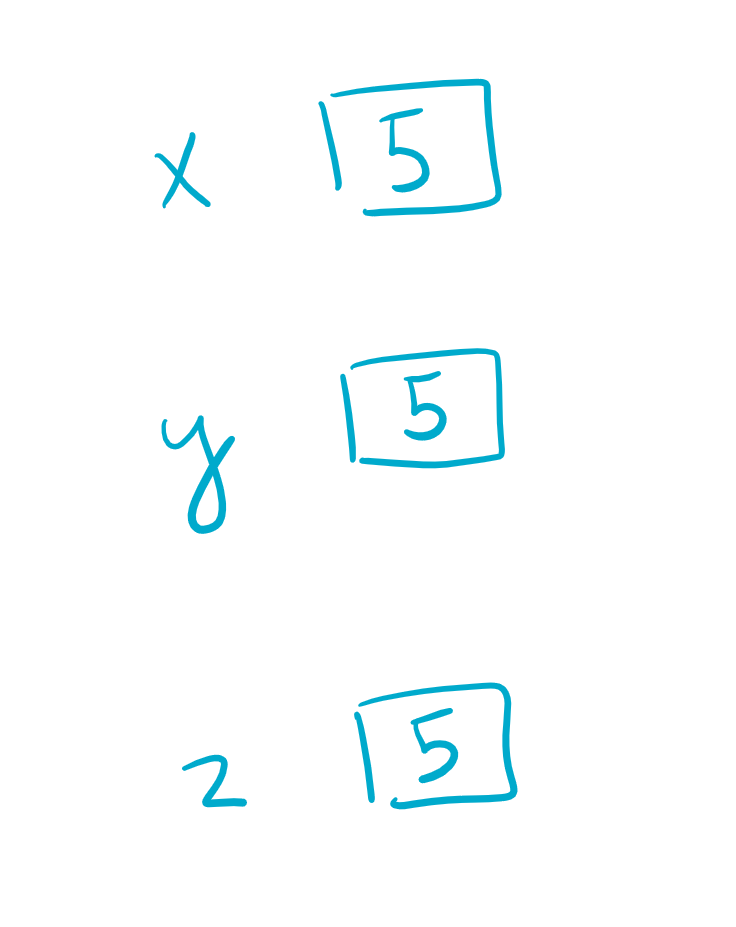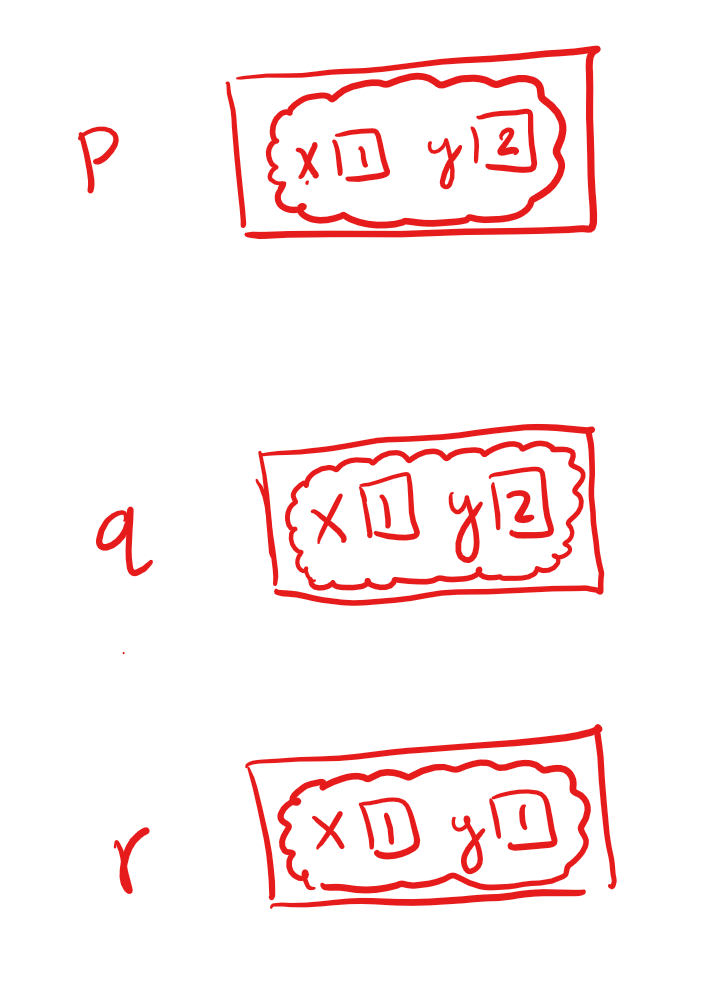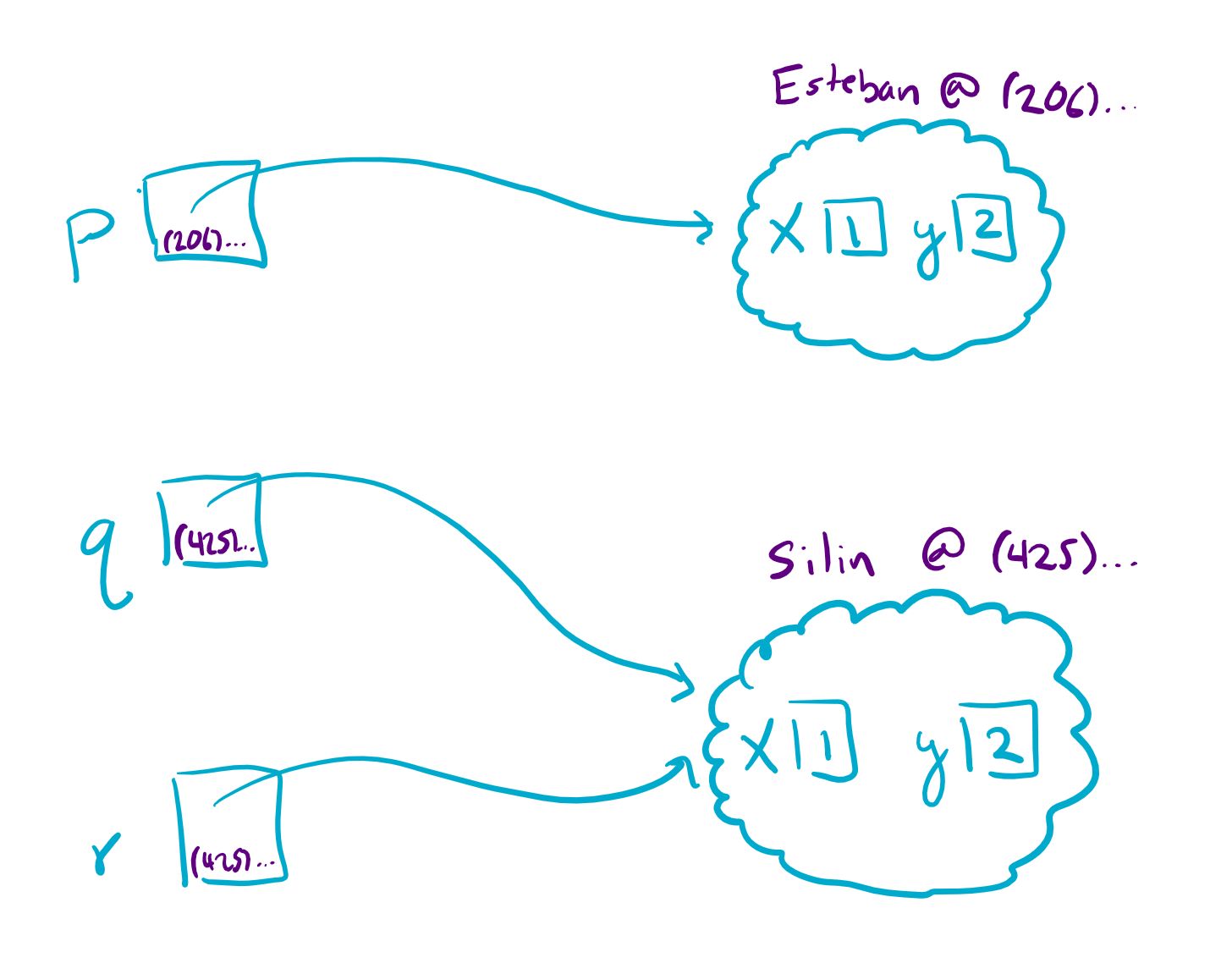## Reference Semantics¶

For your next programming assignment, you will be working with many objects so we wanted to spend today’s reading reviewing reference semantics. It’s really important that you have the correct mental model of how Java stores information in order to understand what is going on in many of the programs we write in 143.

### A more primitive time¶

When you write the lines of code

``````int x = 5;
int y = 5;
int z = y;
``````

You should imagine having three boxes to store values (named x, y, z respectively) and in this case all the values are `5`.It’s important to note when you write `z = y` that you are not somehow linking the variables `z` and `y`. To lay out the steps of how assignment works in Java in detail * Evaluate the right hand side of the assignment. * It is a variable, so look in the box named `y` and use the value `5` inside the box. * Store the number `5` in a new box labeled `z`.

This means when you later say

``````y = y + 1;
``````

You first evaluate the right hand side by looking inside the current value of `y` (which is `5`), add `1` to it (to get `6`), and then store that value in the box called `y`. Note that `z` doesn’t change because we never did an assignment to the `z` variable!

### Now again with objects¶

Imagine the `Point` class

``````public class Point {
private int x;
private int y;

public Point(int x, int y) {
// Hunter: We'll discuss why this is necessary on Friday
this.x = x;
this.y = y;
}

public int getX() {
return x;
}

public int getY() {
return y;
}

public void setX(int x) {
this.x = x;
}

public void setY(int y) {
this.y = y;
}
}
``````

Say I had a similar code snippet as before

``````Point p = new Point(1, 2);
Point q = new Point(1, 2);
Point r = q;
``````

Many people have the wrong picture in their head when thinking about this code! I have shown the wrong picture in red below. The key misunderstanding here is the idea that the `Point` somehow fits inside the variable `p`.`p` does not store the `Point` itself, but rather stores a reference to the `Point` object. The appropriate picture is shown below. You should think of each new object in the program as a person with a phone-number to reach them (shown in the picture in purple). You can think of the variables `p`, `q`, and `r` just storing the phone-numbers so they know who they are talking to.This means when we ran the code `r = q`, just like in the `int` example, we did not somehow make a magical link between the variables `q` and `r`. The steps for how this assignment statement works are exactly the same as they were with primitive variables, with the key distinction that the contents of the box `q` are different than a simple `int`. What is the contents of the box `q`? A reference (or phone-number) to a `Point` object. It’s worth repeating:

Reminder

When you run `r = q`, you don’t copy the object, you only copy the phone-number

Now consider if we had the line

``````q = new Point(q.getX() + 1, q.getY() + 1)
``````

Just like with the `int`s, you first evaluate the right hand side to make a new `Point`. How do you get the values of its `x` and `y`? You first have to call up `q` and ask it what its `x` and `y` are by calling the methods `getX` and `getY`. Think of the line `q.getX()` as calling up the phone-number stored in `q` and asking it to do the `getX` routine. After we compute those values, we create the new `Point` and store its phone-number in `q`. Notice just like last time `r` never changed and it still holds the old phone-number as before.

This idea of reference semantics was why, when we discussed Stacks and Queues, changing the Queue inside the method also changed it in the `main` method.

## Arrays of Objects¶

When you make an array, it initializes all the values to the default value or “zero equivalent of the type”

``````int[] nums = new int;
System.out.println(Arrays.toString(nums)); // [0, 0, 0, 0, 0]
``````

What about if you make an array of `Point`?

``````Point[] points = new Point;
System.out.println(Arrays.toString(points)); // [null, null, null, null, null]
``````

It prints out a bunch of `null` values! It turns out the default value for all reference types is this magic value called `null`.

### What does null mean?¶

Some might think of `null` as an object that doesn’t exist. I think it’s easier to think of in the phone-number analogy as a special phone-number (maybe call it the number `(000) 000-0000`) that no person is allowed to have. This means when you see this special phone-number, you know that there is no associated object (i.e. it’s a dead-end).

It’s totally okay to have a variable store the value `null`! The problem comes up when you call a method on a `null` reference. Imagine we wrote

``````points.setX(3);
``````

This involves getting the phone-number for the first `Point` (which is `null`), and calling up that phone-number to ask it to `setX`. What happens if you call the phone-number `null`? The program crashes with a `NullPointerException`! Java doesn’t want you calling phone-numbers for people that don’t exist and will crash the program.

### How do we fix this?¶

Essentially, you have to go through and set up all the objects in the array explicitly by calling `new`. For example

``````for (int i = 0; i < points.length; i++) {
points[i] = new Point(0, 0);
}
``````

Now the array will store references to 5 new `Point` objects and we can manipulate as we see fit!